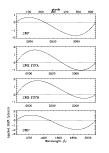Next: 7.2 Additional Corrections for Up: 7.1 Corrections Common to Previous: 7.1.2 Image Rotation

7.1.3 Wavelength Linearization

As discussed in Chapter 8.1, the dispersion solutions for the SI are not precisely linear; significant second- and third-order terms are present in both dispersion modes. The nonlinearities which exist in the dispersion solutions for the SI can, if not accounted for, lead to wavelength errors on the order of several Ångstroms (low dispersion) or several kilometers per second (high dispersion) in some regions of the spectrum. Therefore, a remapping of the SI data is necessary to force the dispersion solution onto a linear scale. In low dispersion, the high-order terms for a given camera are quite stable over time and camera temperature (THDA) so that a single third-order correction can be applied to all of the data for a given camera. The same holds true for high dispersion except that individual solutions are applied independently to each echelle order. The derivation of the corrections is discussed in detail in Chapter 8.1.

The form of the low-dispersion linearization correction for each camera is displayed in Figure 7.1. This figure shows the amount by which each pixel in the low-dispersion SI must be shifted along the dispersion direction from its original uncorrected position in order to achieve a linear dispersion solution. This correction is applied uniformly throughout the spatial direction of the image so that entire image columns (i.e., lines of constant wavelength) are shifted by the same amount.Figure 7.1:  Low-dispersion wavelength linearization correction vectors.

In high dispersion, since the vectors which correct for wavelength nonlinearities are determined separately for each order, the corrections for each order are applied in a ``block-like'' fashion. That is, each pixel in a line of constant wavelength for a given order is shifted by the same amount. The starting and ending spatial positions for each ``block'' about an order are set halfway between the adjacent orders.Next: 7.2 Additional Corrections for Up: 7.1 Corrections Common to Previous: 7.1.2 Image Rotation
Karen Levay
12/4/1997